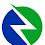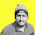### Battery pack capacity calculation for EVs as per our required range.

BY    AFZAL YOUSAF

Battery pack is the motive source in electric vehicles. Designing of battery pack is one the important section in EV Designing and battery pack calculation depends on several factors. Normally range of the vehicle and Motor specfications directly influences the battery pack capacity.

In this article you can get an idea of how to Design/ Calculate battery pack for EV as per your range requirement.

Before designing a battery pack, Let's look the basic parameters of battery.

• Cell voltage - potential difference between the cell terminals. It is differentiated into Max voltage, Min voltage and Nominal voltage. Usually nominal voltage is taken for calculation and design as it is mid range between maximum and minimum voltage.
• Amp hour rating - Amp hour rating of a cell/battery is how much amperage a battery/cell can provide for exactly one hourThe C rating tells you how many amp hours the battery/cell can provide for a very specific period of time.
• C rate - Charging and Discharging rate of cell/battery. Usually it is the rating of the battery capacity. If 100 Ah battery pack has c rate 1C, meaning that a fully charged battery rated at 100 Ah should provide 100 A for one hour. Same battery discharging at 2C, it delivers 200 A for 30 minutes.

Let's consider, we are using a BLDC motor, it draws different amount of current from the battery depending on the speed. Factors we need to consider while designing a battery pack are:-

• Motor power and voltage.
• Gross weight of the vehicle.(Used in selection of Motor)
• Top speed.
• Expected range.
Here we are trying to find the battery pack capacity of a vehicle with gross weight of 250 Kg.  And we are using a 1000 W(Rated) 60 V BLDC Motor to drive the vehicle. We have taken this motor to show an example of how to calculate battery pack capacity. Proper motor selection can only be done after considering parameters like Gross weight of vehicle, Top speed, Drag force, Rolling resistance, Grade, Required acceleration and Regenerative parameters.

After selecting the motor we need to decide the range of the vehicle, and here we are designing a battery pack for a range of 200 KM.

Maximum speed we are aiming is 50 kmph and current drawn by the motor in this speed is calculated below.

Using equation   V*AH/1000 = Motor capacity( in KW) , here Voltage of motor is 60 V, Motor capacity is 1 KW(1000W). So by equating these values, we get 60*AH/1000=1. From these we get AH is 16.6 Amp.

After finding current drawn by the motor, we need to consider Acceleration current. While accelerating motor draws more current and in deceleration draws less. So in this condition some current loss will be there and we take that loss as acceleration current. Here we take it 5% (You can take 5-10% range if your vehicle runs in heavy traffic/or speed of the vehicle varies continuously) .

So by considering acceleration current, effective current drawn by the motor is :-

16.6*1.05(5%) = 17.43 approx 17.5 amp. (In practical tests current drawn will vary slightly but overall will be same)

Then we know Power = V*I.  Power required at 50 kmph speed is 60*17.5 = 1050 Watt.

After finding Power, Here we are designing battery pack for 200 km range and for that we need to consider a Travel factor.

Travel factor = Total range(travel)/ total speed.

Our total range is 200 KM and Total speed is 50 kmph as mentioned above.

So here Travel factor will be 200/50 = 4.  This travel factor is to travel 200 km at 50 kmph speed constantly.  So Power required for it will be Power* Travel factor ie; 1050*4 = 4200 Watt.

So Total power required will be 4.2 Kw.

Then we need to consider the Efficiency of the battery pack. Li-ion Batteries we buy from the market usually have around 85% - 93% charging and discharging efficiency. Considering the worst case, let us take the efficiency of Li-ion battery pack as 85%.

So, Charge/ Discharge efficiency of the battery = 85%

Total Power = 4.2 Kw

So Battery Pack Capacity required = 4.2/0.85 = 4.94 kWh.

Finally we need to consider the Efficiency of BLDC Motor and usually it comes around 85 - 90%. Here we consider BLDC Motor is 85% efficient.

So Total Battery capacity required is 4.94/0.85 =   5.81 kWh.

On a round figure we can conclude that total battery pack capacity required to run a vehicle of 1 KW 60 V motor with 50 kmph speed for 200 KM is 5.85 kWh.

This is how we theoretically calculate the battery pack required for our EV. This will give you a basic idea of calculating your required battery pack. In practical cases, so many other losses will be there and need to have proper testing and evaluation to finalise exact pack value.

Things to remember are:-

• For different speed, motor efficiency will be different because of different RPM.
• When speed varies battery pack capacity also varies. Least requirement of battery pack capacity comes when speed matches the efficiency of the motor we used.
• For eg; For 1 KW BLDC motor we took, efficient speed will be 30-35 kmph and if we design battery pack keeping that speed as maximum, our required battery pack capacity will be less.
• Battery pack capacity also varies depending on motor voltage.
• If gross weight of the vehicle increases, motor capacity increases and gradually battery capacity also increases.
• Need to study the motor performance curve for better calculation.
Subscribe to us to get the notification for article related to  Motor selection.

1.Common hybrid solar inverters, also known as multi-mode inverters, are at the heart of any simple and cost-effective home battery storage system. Hybrid inverters are basically a solar inverter with built-in inverter / battery charger. These modern inverters use energy meters and software that can be programmed to determine the most efficient use of the energy available to you. Learn more about hybrid converters here.

Home off-grid and battery energy storage systems can be divided into four categories below:

Multi-mode off-grid and hybrid inverters

BESS All-in-One Battery Energy Storage System

Hybrid solar power transformers

Battery Systems - Energy Storage

Hybrid transformers are the simplest and most economical way to add a storage battery but it does have some limitations, the most important of which is the limited increase or peak power output. Some hybrid transformers do not have backup power capacity or standby power is very limited, so only small (core) loads can be backed up in In case of power failure. To add a battery to one of the current solar installations, there are several hybrid inverters combined with AC. Solar power panels and inverters do not need external batteries for the system Turkish industry A five-year warranty Application:
1- Green energy systems (solar energy, wind energy, hydro power, etc.)
2- Solar power stations
3. UPS systems
4- Electric Power Supply Systems (EPS)
5- Communications installations
6- Boats or buoys
7. Pointing station
8 Surveying and mapping system 9 / emergency lighting
10- Traffic lights
11- Street lighting required agents in the Arab countries +905346448537 Contact us via Whatsapp for serious inquiries

2.how calculate this and where from this value 16.6*1.05..?

1.16.6*1.05 is the effective current drawn by the motor considering the loss due to accelerating current. 5% is taken as accelerating current loss and it is added with motor current. 1.05 is used to add 5% loss with motor current.
You can also do this by adding 5% of 16.6.
ie; 16.6*(5/100)=0.83. So effective current drawn by motor will be 16.6+0.83 = 17.43. Both calculation is same.

3.1)Is this calculation for E-bikes or all EV's
2)It is assumed that 1kw motor will give 50kmph but then can 2kw an 2.5kw give more top speed?

4.from your calculation point of view mahindra treo zor gives 125km range with 50kmph and motor 7.45kw,48v we calculate as per your data we get 22.9kwh but they use 7.37kwh why these type of difference
one thing if we reduce speed to 25kmph means also we get 46kwh
kindly suggest my calculation is wrong or i missed anything
kindly you recalculate with this data and clear my doubts
Thank you